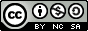## Wednesday, December 26, 2007

### Simple Factorial Generation - Perl versus Bash

Hey there,

I've seen this floating around the boards, so I thought I'd add my 2 cents. Lots of folks (more homework? When will it end?) are looking for scripts to help them find the factorial of any given number.

For those of you who may not know, the factorial of a number is the number itself multiplied by all the numbers from 1 up to that number. So, the factorial of 3 is: 1 times 2 times 3 = 6

Some of the scripts I see are severely convoluted, so I thought I'd put this up here as a little homework help. It can be solved with Perl in 10 lines (Could be less if I wasn't so hung up on formatting ;)

Interestingly enough - it can be done with the same amount of lines in Linux's bash shell, like so (assuming a recursive function). Or, as I wrote in a previous post, you "could" do it in 1 ;)

`factorial ()  {local number=\$1if [ "\$number" -eq 0 ]then    factorial=1else    let "next = number - 1"    factorial \$next    let "factorial = \$number * \$?"fireturn \$factorial}``#!/usr/bin/perl## 2007 - Mike Golvach - eggi@comcast.net## Creative Commons Attribution-Noncommercial-Share Alike 3.0 United States License#print "factorial of: ";chomp(\$factorial = <STDIN>);\$number = \$factorial;if ( \$factorial == 0 ) { \$factorial = 1;}for ( \$factor = \$factorial - 1; \$factor >= 1; --\$factor ) { \$factorial *= \$factor;}printf("Factorial of %d is : %g\n", \$number, \$factorial);`

Enjoy,

, Mike

l